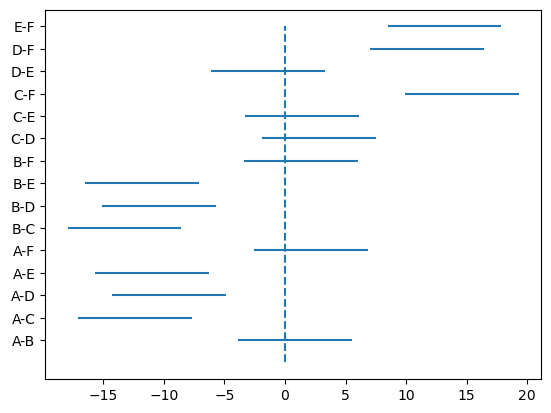# How to perform post-hoc analysis with Tukey’s HSD test (in Python, using statsmodels and Matplotlib)

See all solutions.

If we run a one-way ANOVA test and find that there is a significant difference between population means, we might want to know which means are actually different from each other. One way to do so is with Tukey’s Honestly Significant Differences (HSD) method. It creates confidence intervals for each pair of samples, while controlling for Type I error rate across all pairs. Thus the resulting intervals are a little wider than those produced using Fisher’s LSD method. How do we make these confidence intervals, with an appropriate visualization?

## Solution

We load here the same data that appears in the solution for how to perform pairwise comparisons. That solution used ANOVA to determine which pairs of groups have significant differences in their means; follow its link for more details.

1
2
3
from rdatasets import data
df = data('InsectSprays')
df

count spray
0 10 A
1 7 A
2 20 A
3 14 A
4 14 A
... ... ...
67 10 F
68 26 F
69 26 F
70 24 F
71 13 F

72 rows × 2 columns

We now want to perform an unplanned comparison test on the data to determine the magnitudes of the differences between pairs of groups. We do this by applying Tukey’s HSD approach to perform pairwise comparisons and generate confidence intervals that maintain a specified experiment-wide error rate. Before that, the pairwise_tukeyhsd module needs to be imported from the statsmodels package.

1
2
3
from statsmodels.stats.multicomp import pairwise_tukeyhsd
tukey = pairwise_tukeyhsd(endog=df['count'], groups=df['spray'], alpha=0.05)
print(tukey)

1
2
3
4
5
6
7
8
9
10
11
12
13
14
15
16
17
18
19
20
Multiple Comparison of Means - Tukey HSD, FWER=0.05
=====================================================
group1 group2 meandiff p-adj   lower    upper  reject
-----------------------------------------------------
A      B   0.8333 0.9952  -3.8661  5.5327  False
A      C -12.4167    0.0 -17.1161 -7.7173   True
A      D  -9.5833    0.0 -14.2827 -4.8839   True
A      E    -11.0    0.0 -15.6994 -6.3006   True
A      F   2.1667 0.7542  -2.5327  6.8661  False
B      C   -13.25    0.0 -17.9494 -8.5506   True
B      D -10.4167    0.0 -15.1161 -5.7173   True
B      E -11.8333    0.0 -16.5327 -7.1339   True
B      F   1.3333 0.9603  -3.3661  6.0327  False
C      D   2.8333 0.4921  -1.8661  7.5327  False
C      E   1.4167 0.9489  -3.2827  6.1161  False
C      F  14.5833    0.0   9.8839 19.2827   True
D      E  -1.4167 0.9489  -6.1161  3.2827  False
D      F    11.75    0.0   7.0506 16.4494   True
E      F  13.1667    0.0   8.4673 17.8661   True
-----------------------------------------------------


Because the above table contains a lot of information, it’s often helpful to visualize these intervals. Python’s statsmodels package does not have a built-in way to do so, but we can create our own as follows.

1
2
3
4
5
6
7
import matplotlib.pyplot as plt
rows = tukey.summary().data[1:]
plt.hlines( range(len(rows)), [row for row in rows], [row for row in rows] )
plt.vlines( 0, -1, len( rows )-1, linestyles='dashed' )
plt.gca().set_yticks( range( len( rows ) ) )
plt.gca().set_yticklabels( [ f'{x}-{x}' for x in rows ] )
plt.show()Confidence intervals that cross the vertical, dashed line at $x=0$ are those in which the means across those groups may be equal. Other intervals have mean differences whose 95% confidence intervals do not include zero.